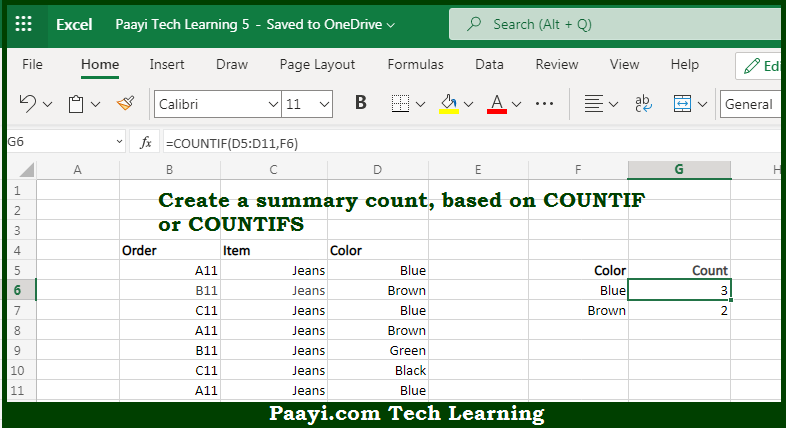# Learn How to Create Summary COUNT With COUNTIF in Microsoft Excel

Written by | 0 Comments | 391 Views

In this article, you will learn how to COUNT various things in Microsoft Excel using a single or combination of functions and its purpose. You will also get to know how to Create a Summary COUNT With COUNTIF and see the generic formula.

Create Summary COUNT With COUNTIF in Microsoft Excel

The main purpose of this formula is to create a summary count. Here we will learn how to create a summary count using a COUNTIF function in Microsoft Excel. That implies, with the help of a formula based on the COUNTIF and COUNTIFS function you can able to create a summary count. So, with the help of this formula, you can able to create a summary count using a COUNTIF function in Microsoft Excel.

General Formula to Create Summary COUNT With COUNTIF

=COUNTIF(range,criteria)

The Explanation for the Create Summary COUNT With COUNTIFSo we know that with the help of the given formula above you can able to create a summary count. Here we will learn how to create a summary count using a COUNTIF function in Microsoft Excel. As we know that the while working with data, a common need is to perform summary calculations that will really generate total counts in different ways. Let's put it like this, total counts by category, color, size, status, etc. The COUNTIF function is a good way to generate these kinds of totals. So, with the help of this formula, you can able to create a summary count using a COUNTIF function in Microsoft Excel.